GFG App
Open AppBrowser
Continue

# Types of Friction – Definition, Static, Kinetic, Rolling and Fluid Friction

Frictional forces are in almost every aspect of the physical world. These forces oppose the motion of the object against each other. In this way, they are both responsible for the motion and resisting the motion. The main reason why these forces occur is due to adhesion. Adhesion is the force of attraction between different bodies. Due to this force, it’s hard to rub different objects against each other and a force resisting this kind of motion is experienced. This is called Friction. Let’s look at these forces in detail.

### Friction

Friction force can be defined as the force generated between two surfaces that come in contact and slide against each other. These forces depend on texture and force applied to them to make them slide against each other. Friction is generated due to the irregularities in the surfaces. Shearing across these irregularities produces this force, which makes the motion harder.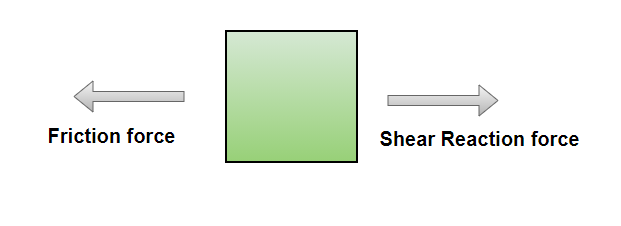If an object is simply placed on another object and moved, the friction will simply be the weight of the object. When the normal force on the surface between two objects starts increasing, the friction also starts increasing. This means that the friction is dependent on the normal force between the surfaces. The maximum amount of force that a surface can apply is given by,

F = μFnorm

The μ mentioned above is called the coefficient of friction. Its value lies between 0 and 1. The frictional force acting on the body when the body is at rest is called static friction, while the frictional force acting on the body when it is moving is called kinetic friction.

### Types of Friction

Friction is a force that resists motion between any two objects. Frictional forces can be divided into multiple categories based on their nature and the materials they act on. Certain frictions only act on solids, while other frictions act between gases and liquids. All these frictions can be divided and sub-divided further. Frictions can be divided into sub-categories as given below:

1. Dry-Friction
• Static Friction
• Kinetic Friction
• Rolling Friction
2. Fluid Friction

### Dry-Friction

Dry friction usually works between solid surfaces. This kind of friction always opposes the movement between two surfaces. This kind of friction is also termed columbic friction. This kind of friction can further be divided into two types – static friction and kinetic friction. The figure below represents the friction acting on the box when a force is applied to it to move it. The force is increased until the box starts moving.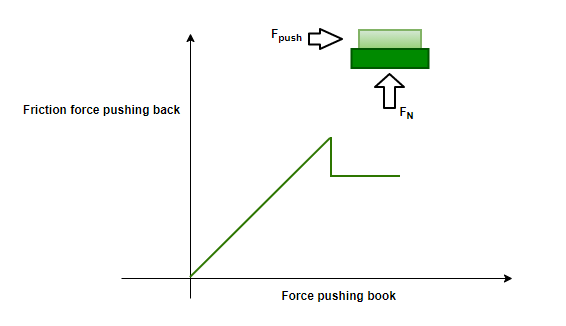Static Friction — This friction before the box starts moving. In this case, the friction is equal to the force applied. As the applied force increases, so does the friction. If the force keeps on increasing, the friction also increases, but there comes a point when the box starts moving. The point just before the box slips is called impeding motion. At this point, the static friction is maximum. It is determined by the coefficient of static frictionFormula for static friction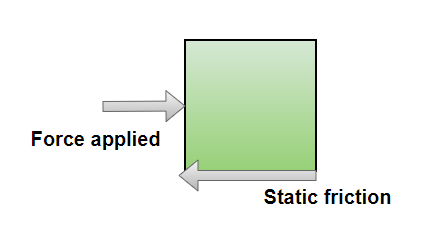Kinetic Friction — Kinetic friction starts acting when the box starts moving. Its value is less than the maximum static friction. In this case, no matter what the applied force is, the value of the kinetic friction will remain the same. In this case, it is determined by the coefficient of kinetic frictionFormula for Kinetic friction: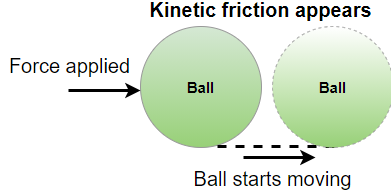Rolling Friction — This happens when a disc or ball rolls over a surface. The reason for this seems to be the distribution of energy involved in the twisting of objects. The coefficient used for the Rolling friction is determined as Crr and is known as Dimensionless rolling resistance coefficient.

Formula for Rolling friction:

F= Crr N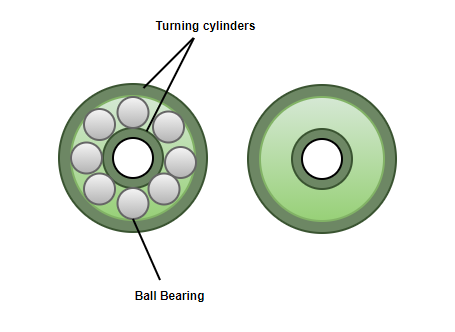Examples —  basketball rolling on the floor, painting the walls using paint rollers, ball bearing (as shown in the figure above).

### Fluid-Friction

This function takes place between fluid layers which move opposite to each other. This friction is internal to the flow and is also called viscosity. Almost all the fluids show resistance to the shearing and are therefore viscous. An airplane flying in the air experiences a drag. This drag is due to the viscosity of the air. Another example is the slowing down of parachutes during sky-diving.

Formula for Fluid friction:A= Area= Difference between the speed of the layersSpacing of the plates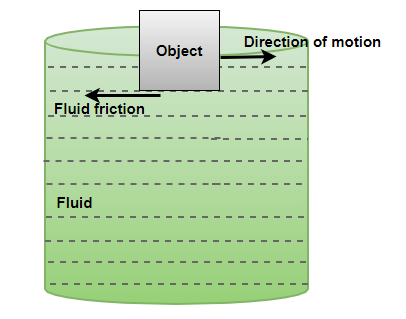### Sample Problems

Question 1: A force of 60N is applied on a 4Kg block kept on the ground. Find the limiting value of static friction if the coefficient of static friction is given by, μs =0.4.

The formula for limiting static friction is given by,Normal Force N = 60 + 4(10) = 100 N

Plugging in the values in the equation,⇒ F = (0.4)(100)

⇒ F = 40N

Question 2: A 4Kg block is kept on the ground. Find the limiting value of static friction if the coefficient of static friction is given by, μs =0.2.

The formula for limiting static friction is given by,Normal Force N = 4(10) = 40 N

Plugging in the values in the equation,⇒ F = (0.2)(40)

⇒ F = 8 N

Question 3: A 4Kg block is kept on the ground. A force of 100N is applied horizontally and the coefficient of kinetic friction is given by,Find the acceleration of the block.

The formula for limiting static friction is given by,Normal Force N = 4(10) = 40 N

Plugging in the values in the equation,⇒ F = (0.2)(40)

⇒ F = 8 N

The kinetic friction will be, 8 N.

Net resultant force = 100 – 8

= 92 N

F = ma

⇒ 92 = 4(a)

⇒ 23 m/s2 = a

Question 4: A 5Kg block is kept on the ground. A force of 10N is applied horizontally and the coefficient of kinetic friction is given by, μs =0.1. Find the acceleration of the block.

The formula for limiting static friction is given by,Normal Force N = 5(10) = 50 N

Plugging in the values in the equation,⇒ F = (0.1)(50)

⇒ F = 5 N

The kinetic friction will be, 5 N.

Net resultant force = 10 – 5

= 5 N

F = ma

⇒ 5 = 5(a)

⇒ 1 m/s2 = a

Question 4: A 5Kg block is kept on the ground. A force of 10N is applied horizontally and the coefficient of kinetic friction is given by,. Find the acceleration.

The formula for limiting static friction is given by,Normal Force N = 5(10) = 50 N

Plugging in the values in the equation,⇒ F = (0.1)(50)

⇒ F = 5 N

The kinetic friction will be, 5 N.

Net resultant force = 10 – 5

= 5 N

F = ma

⇒ 5 = 5(a)

⇒ a= 1 m/sec2

My Personal Notes arrow_drop_up